NEET  >  NEET Previous Year Questions (2014-21): Solutions

# NEET Previous Year Questions (2014-21): Solutions Notes | Study Chemistry Class 12 - NEET

## Document Description: NEET Previous Year Questions (2014-21): Solutions for NEET 2022 is part of Chemistry Class 12 preparation. The notes and questions for NEET Previous Year Questions (2014-21): Solutions have been prepared according to the NEET exam syllabus. Information about NEET Previous Year Questions (2014-21): Solutions covers topics like and NEET Previous Year Questions (2014-21): Solutions Example, for NEET 2022 Exam. Find important definitions, questions, notes, meanings, examples, exercises and tests below for NEET Previous Year Questions (2014-21): Solutions.

Introduction of NEET Previous Year Questions (2014-21): Solutions in English is available as part of our Chemistry Class 12 for NEET & NEET Previous Year Questions (2014-21): Solutions in Hindi for Chemistry Class 12 course. Download more important topics related with notes, lectures and mock test series for NEET Exam by signing up for free. NEET: NEET Previous Year Questions (2014-21): Solutions Notes | Study Chemistry Class 12 - NEET
 1 Crore+ students have signed up on EduRev. Have you?

Q.1. The following solutions were prepared by dissolving 10g of glucose (C6H12O6) in 250 ml of water (P1). 10g of urea (CH4N2O) in 250 ml of water (P2) and 10 g of sucrose (C12H22O11) in 250 ml of water (P3). The decreasing order of osmotic pressure of these solutions is:     (2021)
(a) P2 > P3 > P1
(b) P3 > P1 > P2
(c) P2 > P1 > P3

(d) P1 > P> P3
Ans:
C
π = iCRT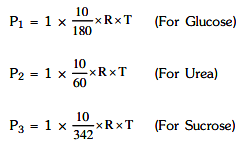∴ P2 > P1 > P3

Q.2. The correct option for the value of vapour pressure of a solution at 45°C with benzene to octane in a molar ratio 3:2 is : [At 45°C vapour pressure of benzene is 280 mm Hg and that of octane is 420 mm Hg. Assume Ideal gas]     (2021)
(a) 336 mm of Hg
(b) 350 mm of Hg
(c) 160 mm of Hg
(d) 168 mm of Hg
Ans:
A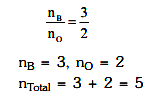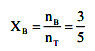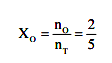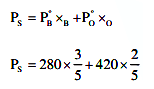= 336 mm of Hg

Q.3. The mixture which shows positive deviation from Raoult’s law is :     (2020)
(a) Acetone + Chloroform
(b) Chloroethane + Bromoethane
(c) Ethanol + Acetone
(d) Benzene + Toluene
Ans:
C
Acetone + ethanol shows positive deviation from Raoult’s law. Pure ethanol possesses H-bonding and adding acetone to ethanol causes breaking of some H-bonds.
This causes increase in observed vapour pressure.

Q.4. The freezing point depression constant (Kf) of benzene is 5.12 K kg mol-1. The freezing point depression for the solution of molality 0.078 m containing a non-electrolyte solute in benzene is (rounded off upto two decimal places) :     (2020)
(a) 0.40 K
(b) 0.60 K
(c) 0.20 K
(d) 0.80 K
Ans:
A
ΔTf = ikfm
⇒ ΔTf = 1 × 5.12 × 0.078
ΔTf = 0.3993
ΔTf = 0.40 K

Q.5. For an ideal solution, the correct option is :    (2019)
A: Δmix S = 0 at constant T and P
B: Δmix V ≠ 0 at constant T and P
C: Δmix H = 0 at constant T and P
D: Δmix G = 0 at constant T and P
Ans:
C
For ideal solution,
Δmix H = 0
Δmix S > 0
Δmix G < 0
Δmix V = 0

Q.6. The mixture that forms maximum boiling azeotrope is:    (2019)
A: Water + Nitric acid
B: Ethanol + Water
C: Acetone + Carbon disulphide
D: Heptane + Octane
Ans:
A
Solutions showing negative deviation from Raoult's law form maximum boiling azeotrope. Water and Nitric acid → forms maximum boiling azeotrope.

Q.7. If molality of the dilute solutions is doubled, the value of molal depression constant (Kf) will be :-    (2017)
A: halved
B: tripled
C: unchanged
D: doubled
Ans:
C
Kdoes not depend on concentration of solution. It only depends on nature of solvent so it will be unchanged.

Q.8. Which of the following is dependent on temperature?    (2017)
A: Molarity
B: Mole fraction
C: Weight percentage
D: Molality
Ans:
A
Temperature dependent unit is molarity.

Q.9. At 100°C the vapour pressure of a solution of 6.5 g of a solute in 100 g water is 732 mm. If Kb = 0.52, the boiling point of this solution will be :    (2016)
A: 103°C
B: 101°C

C: 100°C
D: 102°C
Ans:
B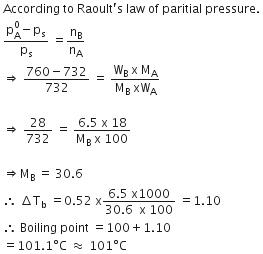Q.10. Which of the following statements about the composition of the vapour over an ideal 1 : 1 molar mixture of benzene and toluene is correct ?
Assume that the temperature is constant at 25°C. (Given Vapour Pressure Data at 25ºC, Benzene = 12.8kPa, toluene = 3.85kPa)    (2016)
A: Not enough information is given to make a prediction.
B: The vapour will contain a higher percentage of benzene.
C: The vapour will contain a higher percentage of toluene.The vapour will contain a higher percentage of toluene.
D: The vapour will contain equal amounts of benzene and toluene.
Ans:
B
Due to high partial vapour pressure of Benzene as compare to that of toluene so the mole fraction of Benzene will be higher than that of toluene. As a result the vapour will contain a higher percentage of Benzene.

Q.11. Which one is not equal to zero for an ideal solution ?    (2015)
A: ΔP = Pobserved - PRaoult
B: Δ Hmix
C: ΔSmix
D: Δ Vmix
Ans:
C
For ideal solution, ΔSmix = 0.

Q.12. The boiling point of 0.2 mol kg-1 solution of X in water is greater than equimolal solution of Y in water. Which one of the following statements is true in this case ?    (2015)
A: Y is undergoing dissociation in water while X under goes no change
B: X is undergoing dissociation in water
C: Molecular mass of X is greater than the molecular mass of Y.
D: Molecular mass of X is less than the molecular mass of Y.
Ans:
B
Molality of solution of x = molality of solution of y = 0.2 mol/kg
By elevation in boiling point relation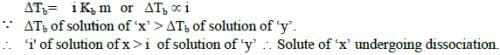Q.13. Which one of the following electrolytes has the same value of van‘t Hoff‘s factor (i) as that of Al2(SO4)3 (if all are 100% ionised)    (2015)
A: K4[Fe(CN)6]
B: K2SO4
C: K3[Fe(CN)6]
D: Al(NO3)3
Ans:
A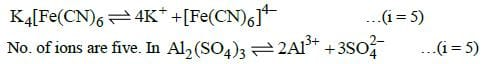Q.14. Of the following 0.10 m aqueous solutions, which one will exhibit the largest freezing point depression?    (2014)
A: Al2 (SO4 )3

B: K2SO4
C: KCl
D: C6H12O6
Ans:
A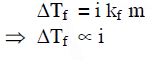The document NEET Previous Year Questions (2014-21): Solutions Notes | Study Chemistry Class 12 - NEET is a part of the NEET Course Chemistry Class 12.
All you need of NEET at this link: NEET

## Chemistry Class 12

157 videos|362 docs|261 tests
 Use Code STAYHOME200 and get INR 200 additional OFF

## Chemistry Class 12

157 videos|362 docs|261 tests

### How to Prepare for NEET

Read our guide to prepare for NEET which is created by Toppers & the best Teachers

Track your progress, build streaks, highlight & save important lessons and more!

,

,

,

,

,

,

,

,

,

,

,

,

,

,

,

,

,

,

,

,

,

;